# Motor Power Calculations

“What are the major criteria by which the performance of an electric motor drive can be evaluated?”

This is a question commonly asked by designers and engineers looking to specify an electric motor for an application. And the answer is pretty straightforward: motor power, speed, efficiency, and torque are vital to the performance of an electric motor for a particular application.

This article presents valuable information about sizing motors for different applications. It will cover design considerations and several calculations, including motor efficiency, torque, and motor power calculations.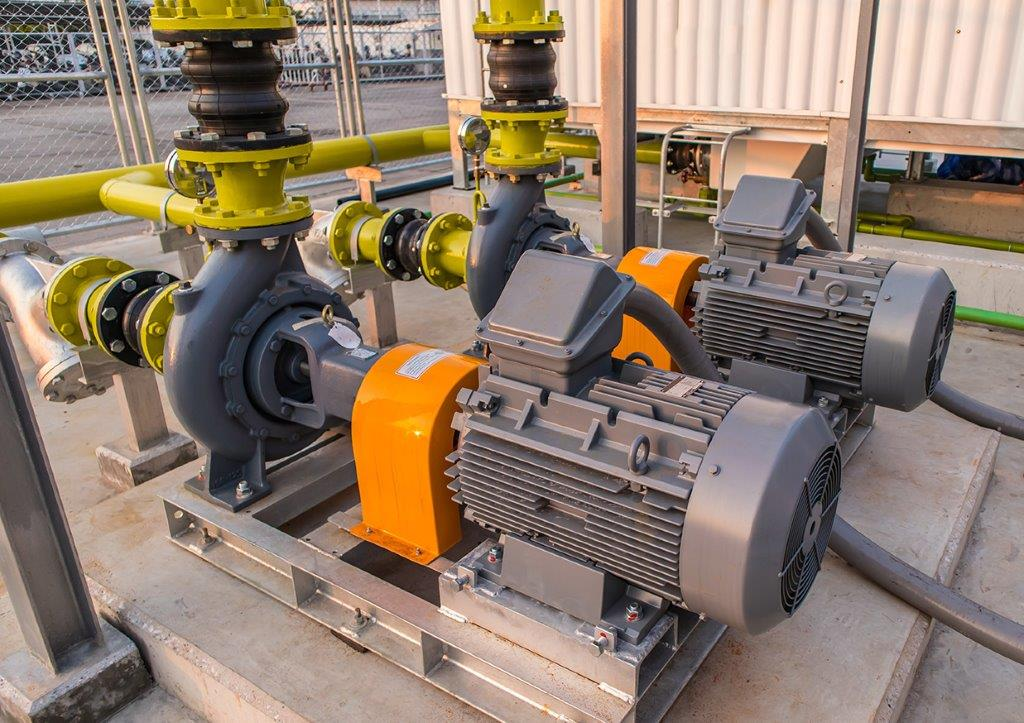Electric motor used for water pump

## How do Electric Motors Work?

Electric motors are designed to convert electrical energy into mechanical energy. Despite the wide range of motor options available today, electric motors typically work based on the magnetic effect of current: an electric current flowing through a wire coil in a magnetic field creates a force that rotates the coil, thus creating torque.

To properly specify electric motors, engineers must understand and know how to calculate the following:

1. Electrical Power Input of the motor
2. Mechanical Power Output of the motor
3. The efficiency of the motor
4. Motor amperage

## Motor Power Calculations (Electric Power Input)

Electric power is defined as the rate at which electrical energy is transferred by an electric circuit. The electric power input of an electric motor can be estimated using:Where:

Pin=Electric power input (Watts or W)

I=Current (A)

V=Applied Voltage (V)

However, keep in mind that the formula above is only applicable to DC sources. Determining the electric power input for an AC source must include a power factor.

Power factor is a measure of how effectively electrical power is being used. It is the ratio of the electrical power that the motor uses (also called real power) compared to the overall amount of power supplied to the circuit (also called apparent power).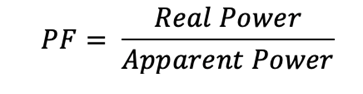The electric power input formula becomes: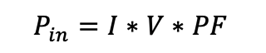A power factor of 1 describes a perfect electric power input system (which is practically impossible to achieve).

## Motor Power Calculations (Mechanical Power Output)

Mechanical power describes the output power that moves the object attached to the motor. It is simply defined as speed times torque (the rotational equivalent of a linear force).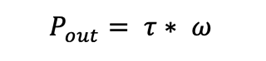Where:

Pout=Output power (W)

t=Torque (Nm)

The angular speed of the motor can be estimated if designers know the rotational speed of the motor, as shown in the equation below: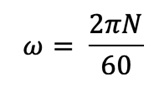Where:

N=Rotational speed (rpm)

## Motor Horsepower Calculations (Mechanical Power Output)

Mechanical power can also be defined in Horsepower (hp). For example, a mechanical power level of 1 hp is equivalent to 746 watts (W) or 0.746 kilowatts. Here is a formula for electric motor horsepower calculations: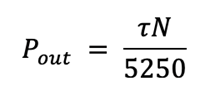Where:

Pout=Output power (hp)

t=Torque (lbf.ft)

N=Rotational speed (rpm)

Engineers can also utilize the electric motor horsepower calculators on manufacturers’ websites for their motor power calculation needs.

## Motor Efficiency Calculator: A Key Formula for Electric Motor Performance

The efficiency of an electric motor is simply the ratio of the mechanical power output to the electric power input.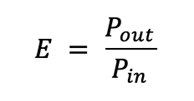Because energy is lost (usually in the form of heat) as an electric motor converts electrical energy into mechanical energy, the mechanical power output is always less than the electric power input. What this means is that motor efficiency will always beless than 1 (or 100%); t ypical electric motor designs have efficiency ranging between70 and 96%.

Consider an electric motor with an input electrical power of 1.36 W that generates a torque of 0.0098 Nm and rotational speed of 1000 rpm. By combining the power input, power output, and efficiency form ula s, the total mechanical power output would be 1.026 W, and the motor efficiency would be 0.7544 (or 75.44%).

This means that approximately three-quarters of the electricity consumed by the motor drive is converted into useful mechanical power , while the remaining one-quarter is lost in the form of heat.

## Motor Full Load Amps Calculators

Amperage (or Amps) is simply the strength of an electric current or the amount of electrical current that flows through an electric conductor in a given time.

The following sections will provide formulas for estimating amperage for single-phase and three-phase systems when mechanical power output (or motor rating) is known.

### 1-Phase Motor Full Load AmpsCalculations

The 1-phase motor full load amps calculations (or single-phase motor FLA calculations) are shown below: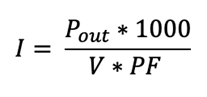Where:

Pout=mechanical power output (kW)

PF=Power Factor

V=Voltage (V)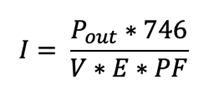Where:

Pout=Mechanical power output (measured in hp)

E=Efficiency

### 3-Phase Motor Amperage Calculator

The 3-phase motor amps calculations are shown below: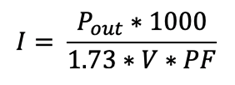Where:

Pout=Mechanical power output (kW)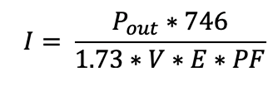Where:

Pout=Mechanical power output (hp)

Engineers can also utilize the 3-phase motor amps calculators (or full load amp calculators) on motor manufacturers’ websites for amperage calculations.

## Electric Motor Calculations: Manufacturers Can Help

While this article provides helpful information about electric motors, there still exist many other factors that engineers must consider when specifying electric motors for a particular application. Therefore, engineers are advised to reach out to manufacturers to discuss their application requirements.